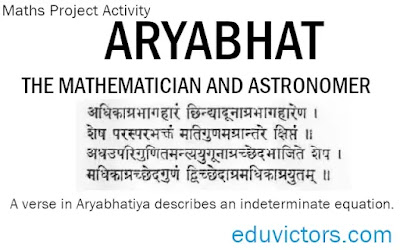# ARYABHAT – THE MATHEMATICIAN AND ASTRONOMER

A brief profile and his contributions

Class 10 Maths Project Activity

Objective: Write about the contributions made by the ancient Indian Mathematician, Aryabhat

## ARYABHAT – THE MATHEMATICIAN AND ASTRONOMER

Life Profile:

One of the Indian mathematicians of ancient times about which some definite information is available is Aryabhat (also called Aryabhata I ).  He was born in 476 AD in Kusumpura (now Patna) in Bihar. Aryabhata wrote many mathematical and astronomical treatise and one of his famous compilation on Mathematics and Astronomy is called Aryabhattiya. He died in 550AD

About Aryabhattiya:

Aryabhattiya is in Sanskrit and is divided into four major parts called 'Pads'. The manuscript contains a total of 153 Shlokas (verses).

1. Its mathematical section, called Dashgeetika Pad (दशगीतिका पद), contains 33 verses giving 66 mathematical rules without proof.

2. Ganitpad (गणित पद)  containing 25 verses

The mathematical part of the Aryabhatiya covers arithmetic, algebra, plane trigonometry and spherical trigonometry. It also contains continued fractions, quadratic equations, sums of power series and a table of sines.

3. Kalkriya Pad (कालक्रिया पद) has 25 shlokas (Time Calculations)

4. Goladhyay (गोलाध्याय) has 50 shlokas (Planet Calculations)

The third and fourth section talks about spheres, ellipses, time and planetary models.

Contributions:

1. Aryabhat created a new method of enumerating numbers using Sanskrit alphabets. According to this, he gave the following numerical values to 25 consonants:

क : 1,  ख : 2,  ग : 3,  घ  : 4,  ङ : 5,

च  : 6, छ : 7,  ज: 8,  झ : 9,  ञ : 10,

ट : 11,  ठ : 12,  ड : 13,  ढ : 14,  ण : 15,

त: 16,  थ: 17,  द  : 18,  ध : 19,  न : 20,

प : 21, फ : 22,  ब  : 23,  भ  : 24,  म  : 25,

य  : 30,   र  : 40,  ल : 50,   व  : 60,  श : 70,

ष  : 80,  स  : 90,  ह : 100

He gave the following values to vowels (स्वर)

अ :  1,       ई   : 100,    ऊ   :  10000

ऋ : 10⁶,    ऌ   : 10⁸,    ए  :  10¹⁰

ऐ  : 10¹²,    ओ  : 10¹⁴,   औ  : 10¹⁶

This shows Aryabhata was also familiar with numeral symbols and the place-value system.

2. Aryabhat has summarised important rules of arithmetic, geometry and algebra in 33 shlokas of Ganitpad only. In these shlokas, he has given formulae for finding:

• squares and square-roots

• cubes and cube-roots

• area of squares, triangles and circles

• volume of a sphere

• table of sines

• summing of first n integers, their squares and cubes

3. Aryabhata gave an accurate approximation for π, correct up to 8 decimal places. He wrote in the Aryabhatiya the following:

‘Add four to one hundred, multiply by eight and then add sixty-two thousand. the result is approximately the circumference of a circle of diameter twenty thousand. By this rule, the relation of the circumference to diameter is given.’

62832

π=    --------  = 3.1416

20000

4. With many examples, he stated and verified Pythagoras theorem.

5. Aryabhat has given methods of drawing a circle, a triangle and quadrilateral and solving of quadratic equations.

6. Another important contribution of Aryabhat has been the formation of tables of sine and cosine functions at intervals of 3°45' each. (90° ÷ 24 = 3°45' ). He also introduced the versine (versin = 1 - cosine) into trigonometry.

7. In Goladhyay, Aryabhat wrote about astronomy.  He calculated the circumference of the earth as 4967 yojanas (= 59604 km  higher than the present estimated value 40,075 km. 1 yojana = 12 kilometres approximately.)

8. He was the first mathematician who declared that the earth revolves about its axis. he stated the correct number of days in a year i.e. 365.

9. He also described solar and lunar eclipses and reasons for their occurring.

10. He believed that the Moon and planets shine by reflected sunlight.

Honours:

In 1954, an institute,  are both named in his honour. An Institute for conducting research in astronomy, astrophysics and atmospheric sciences is the Aryabhatta Research Institute of Observational Sciences,  was set up near Nanital to conduct research in astronomy, astrophysics and atmospheric sciences. The Indian government named its first satellite Aryabhata (launched 1975) in his honour.

👉See Also:

Lab Activity: To verify the distance formula by graphical method#### Post a comment

We love to hear your thoughts about this post!